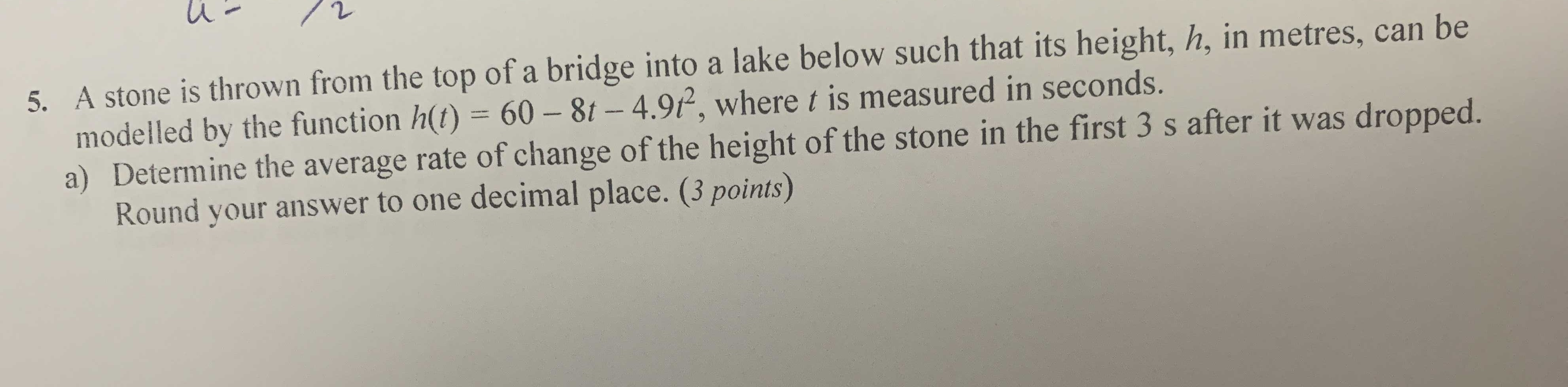### ¿Todavía tienes preguntas de matemáticas?

Pregunte a nuestros tutores expertos
Algebra
Pregunta

5. A stone is thrown from modelled by the function a) Determine the averag Round your answer t A stone is thrown from the top of a bridge into a lake below such that its height, $$h$$ , in metres, can be modelled by the function $$h ( t ) = 60 - 8 t - 4.9 t ^ { 2 }$$ , where $$t$$ is measured in seconds. a) Determine the average rate of change of the height of the stone in the first $$3 s$$ after it was dropped. Round your answer to one decimal place. (3 points)$$h(0)= 60,h(3)= - 8.1\\r= \frac{h(3)- h(0)}{3} = - 22.7$$